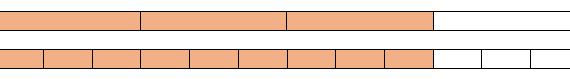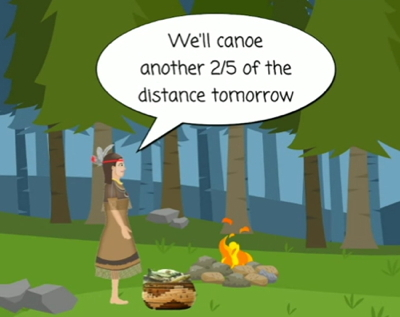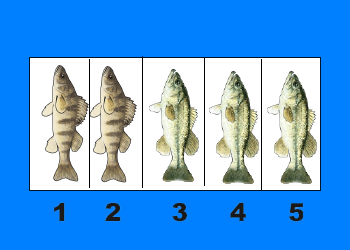# equivalent fractionsToday's post features another fraction problem contributed by a middle school student. This one teaches equivalent fractions and common denominators.

## Part 2: Math Problems and Middle School Game Design ProjectOne way to teach students fractions is to have them write their own problems and give the answer. Next, have them solve and discuss each other's problems. Play the Fish Lake game to get some ideas of fractions problems.

## Math Problems and Middle School Game Design Project: Part 1To add fractions there are three simple steps: Step 1: Make sure the bottom numbers (the denominators) are the same. Step 2: Add the top numbers (the numerators), put that answer over the denominator. Step 3: Simplify the fraction (if needed) We believe that children learn from multiple examples in multiple situations. I’m sure you, oh […]

## Math Lesson – Adding like fractions (with a common denominator)Equivalent fractions are different fractions that name the same number. The numerator and the denominator of a fraction must be multiplied by the same nonzero whole number in order to have equivalent fractions. The presentations and videos below provide multiple explanations and examples. Using Equivalent Fractions on a Number Line I really like this PPT […]